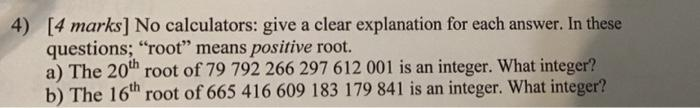# Question Solved1 AnswerPlease show all steps and written explanation for both parts thank you so much have an amazing day 4) [4 marks] No calculators: give a clear explanation for each answer. In these questions; “root” means positive root. a) The 20th root of 79 792 266 297 612 001 is an integer. What integer? b) The 16th root of 665 416 609 183 179 841 is an integer. What integer?Please show all steps and written explanation for both parts thank you so much have an amazing dayTranscribed Image Text: 4) [4 marks] No calculators: give a clear explanation for each answer. In these questions; “root” means positive root. a) The 20th root of 79 792 266 297 612 001 is an integer. What integer? b) The 16th root of 665 416 609 183 179 841 is an integer. What integer?
More
Transcribed Image Text: 4) [4 marks] No calculators: give a clear explanation for each answer. In these questions; “root” means positive root. a) The 20th root of 79 792 266 297 612 001 is an integer. What integer? b) The 16th root of 665 416 609 183 179 841 is an integer. What integer?